## Facial Emotion Recognition using Deep Learning

By: Ram on Nov 24, 2020

Found facial expressions of emotion are not culturally determined, but universal across human cultures and thus biological in origin.

The 6 basic human emotions are anger, disgust, fear, joy, sadness, and surprise. Reference Source.

In this blog, we aim to build a deep learning-based human facial emotion classifier. For building an emotion classifier, we need to have facial expression images and a linked human emotion class.

We have a data sample on Kaggle with the required data. We will use this sample for building CNN based Deep Learning Model which will classify emotion based on facial emotions.

The data file has 3 columns - one emotion category, second the pixels of an image, and third whether the row is for training or testing.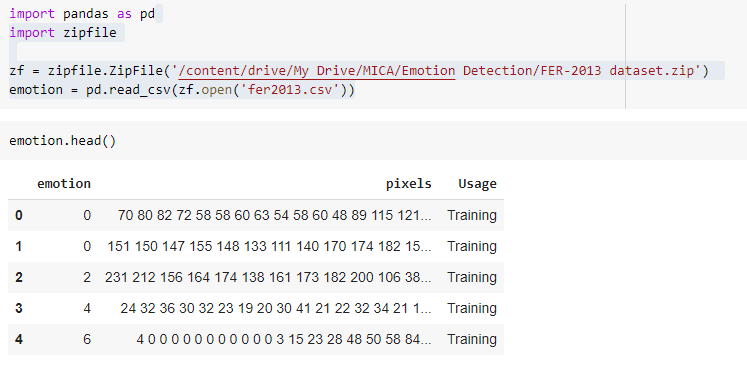If we look at the data frame details, the emotions are categorized as an integer, the images are converted to the pixels and they are available in the pixel's column. Also, the Usage column gives information on whether we can consider row/image for training/test/validation.

We will explore the data further.  We can see the distribution of emotion category.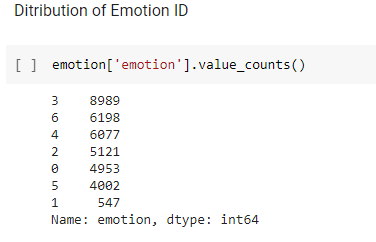We may want to view a few sample images and their emotion ID. Since image pixels are stored in a single column of the data frame. We may want to change it to array format so that we can plot an image based on the image pixels.

`import numpy as np`

`img_pixels =[]`

`for i in range(len(emotion['pixels'])):`

`  pix = np.array(emotion['pixels'][i].split(" "),dtype=float)`

`  pix.shape = (48,48)`

`  img_pixels.append(pix)`

`img_pixels = np.array(img_pixels)`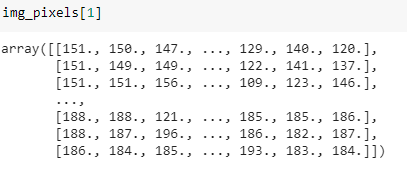`# plot some of the numbers`

`import matplotlib.pyplot as plt`

`plt.figure(figsize=(50,50))`

`for i in range(0,10):`

`    plt.subplot(1,10,i+1)    `

`    plt.imshow(img_pixels[i], interpolation = "none", cmap = "afmhot")`

`    plt.xticks([])`

`    plt.yticks([])`

`    plt.title(str(emotion_labels[emotion['emotion'][i]]))`

`plt.tight_layout()`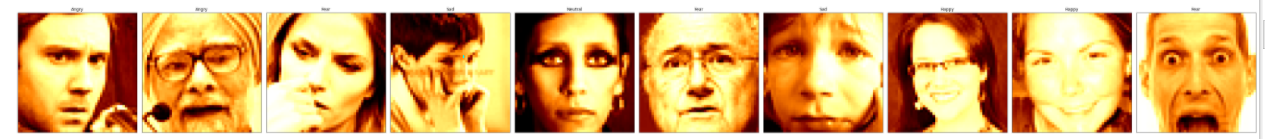### Feature Standardization

Pixel values range between 0 and 254. We can bring to 0 and 1 range. We can use the standard scaler function to do this.

`import numpy as np`

`from sklearn.preprocessing import StandardScaler`

`scalers = {}`

`img_pixels_features= img_pixels`

`for i in range(img_pixels.shape):`

`    scalers[i] = StandardScaler()`

`    img_pixels_features[i, :, :] = scalers[i].fit_transform(img_pixels[i, :, :])`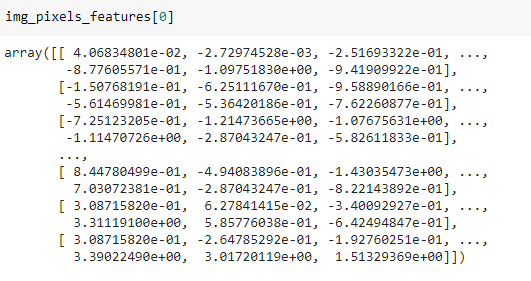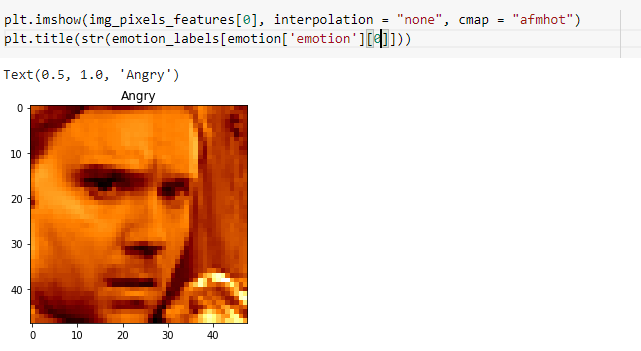Now, we have standardized image arrays ready. We can split the sample into training, test, and validation samples.

### Split into Training and Validation Samples

We already have a column to split the sample into Training, Testing, and Validation Samples.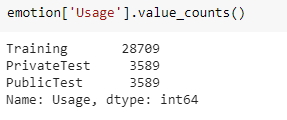### Training Sample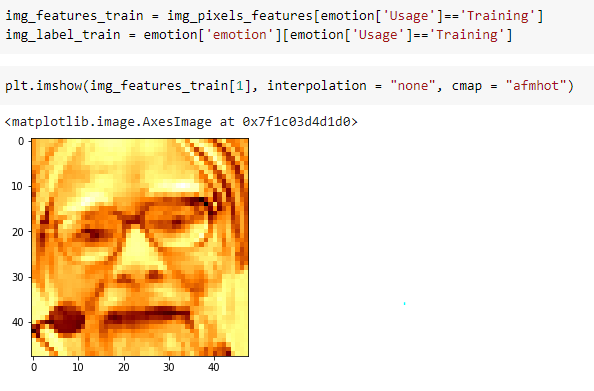Testing Sample – Private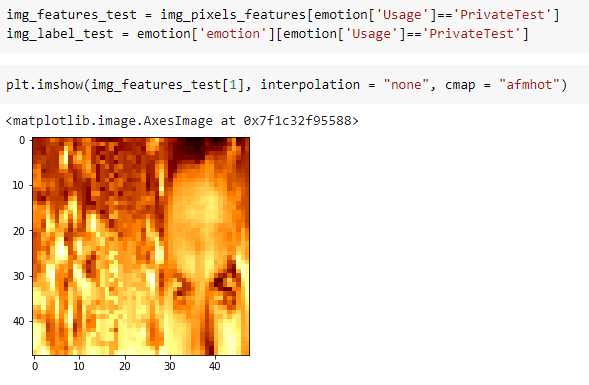from keras.utils import np_utils

img_label_test = np_utils.to_categorical(img_label_test, 7)

print (img_label_test)

### Testing Sample – Public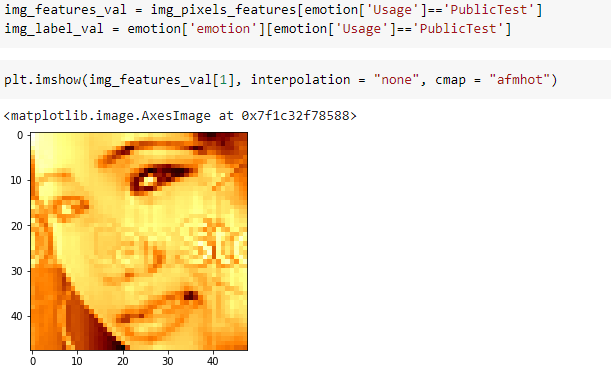### Label – One Hot Encoding

We need to do one-hot encoding of the label – emotion category.

`from keras.utils import np_utils`

`img_label_val= emotion['emotion']`

`img_label_val = np_utils.to_categorical(img_label_val, 7)`

`print (img_label_val)`

### CNN Model Architecture

We need to define a model architecture for the deep learning model which will be used for facial emotion classification. We are using Keras for deep learning architecture.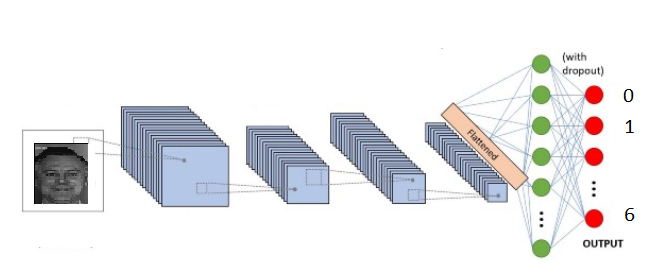`from keras.models import Sequential`

`from keras.layers import Dense , Activation`

`from keras.layers import Dropout`

`from keras.layers import Flatten`

`from keras.constraints import maxnorm`

`from keras.optimizers import SGD , Adam`

`from keras.layers import Conv2D , BatchNormalization`

`from keras.layers import MaxPooling2D`

`from keras.utils import np_utils`

`model = Sequential()`

`model.add(Conv2D(32, (3, 3), activation='relu', padding="same", input_shape=(48,48,1)))`

`model.add(Conv2D(32, (3, 3), padding="same", activation='relu'))`

`model.add(MaxPooling2D(pool_size=(2, 2)))`

`model.add(Conv2D(64, (3, 3), activation='relu', padding="same"))`

`model.add(Conv2D(64, (3, 3), padding="same", activation='relu'))`

`model.add(MaxPooling2D(pool_size=(2, 2)))`

`model.add(Conv2D(96, (3, 3), dilation_rate=(2, 2), activation='relu', padding="same"))`

`model.add(Conv2D(96, (3, 3), padding="valid", activation='relu'))`

`model.add(MaxPooling2D(pool_size=(2, 2)))`

`model.add(Conv2D(128, (3, 3), dilation_rate=(2, 2), activation='relu', padding="same"))`

`model.add(Conv2D(128, (3, 3), padding="valid", activation='relu'))`

`model.add(MaxPooling2D(pool_size=(2, 2)))`

`model.add(Flatten())`

`model.add(Dense(64, activation='sigmoid'))`

`model.add(Dropout(0.4))`

`model.add(Dense(7 , activation='softmax'))`

`Model Compilation`

`model.compile(loss='categorical_crossentropy',`

`              optimizer='adam' ,`

`              metrics=['accuracy'])`

`print(model.summary())`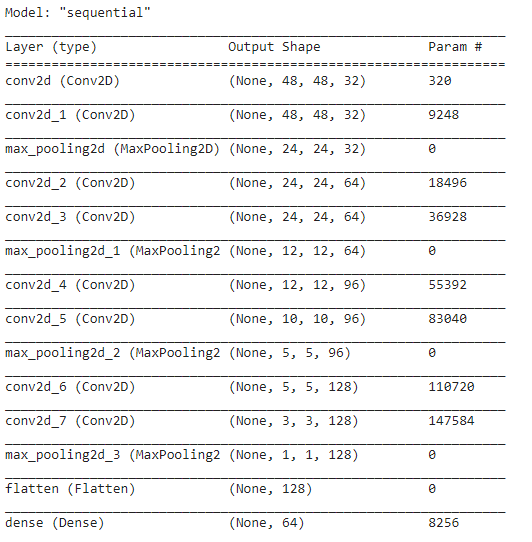### Change the shape of the input array

kX_train = img_features_train.reshape(len(img_features_train),48,48,1)

kX_test = img_features_test.reshape(len(img_features_test),48,48,1)

### Image generations

from keras.preprocessing.image import ImageDataGenerator

datagen = ImageDataGenerator(

featurewise_center=False,

samplewise_center=False,

featurewise_std_normalization=False,

samplewise_std_normalization=False,

zca_whitening=False,

rotation_range=10,

zoom_range = 0.0,

width_shift_range=0.1,

height_shift_range=0.1,

horizontal_flip=False

vertical_flip=False)

datagen.fit(kX_train)

### Model Fitting

Fitting the model based on the train samples.

batch_size = 64

epochs = 30

validation_steps = len((img_features_train, img_label_train)) // batch_size

history = model.fit_generator(datagen.flow(kX_train, img_label_train, batch_size=batch_size),

validation_data=(kX_test, img_label_test),

epochs = epochs, verbose = 2)

We should save the model for future use and we can load the model.

import tensorflow as tf

# We can save our model with:

model.save('emotion_model.h5')

### Model Performance

plt.plot(history.history['accuracy'])

plt.plot(history.history['val_accuracy'])

plt.title('model accuracy')

plt.ylabel('accuracy')

plt.xlabel('epoch')

plt.legend(['train''test'], loc='upper left')

plt.show()

# summarize history for loss

plt.plot(history.history['loss'])

plt.plot(history.history['val_loss'])

plt.title('model loss')

plt.ylabel('loss')

plt.xlabel('epoch')

plt.legend(['train''test'], loc='upper left')

plt.show()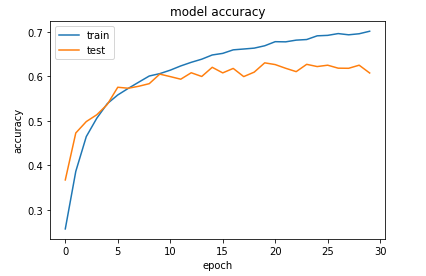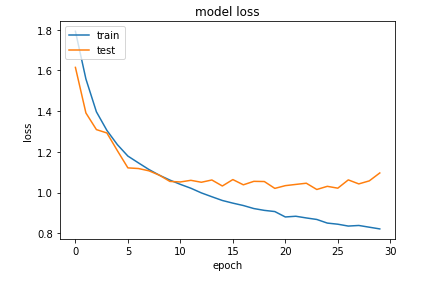Confusion Matrix

Comparing the predicted and actual emotion category.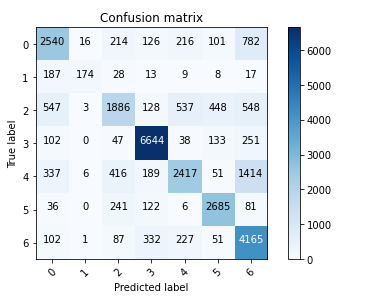It is working fine in some of the emotion categories but not doing a good job in others. We can augment data or use a pre-trained model (transfer learning) to see if that helps us in improving the accuracy of the model.

TagsMost PopularJun 18, 2020Jul 23, 2020Jun 19, 2020Jun 19, 2021